## Two-sided Rounded Tetrahexes

Here is the set of 52 rounded tetrahexes with a total area of 208. The SVG-file of these pieces is here.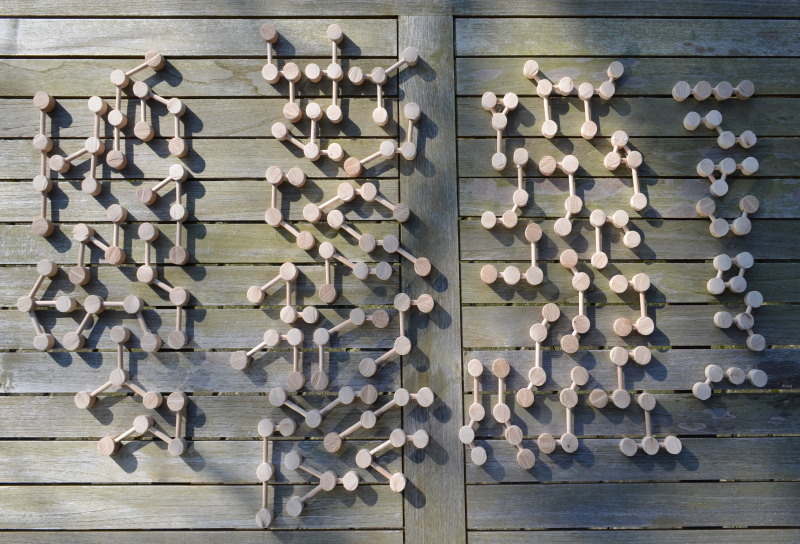A semiregular hexagon made with these pieces is shown in the title and is an example of a figure with triangle symmetry. Figures with one or two axes of symmtry ar shown below.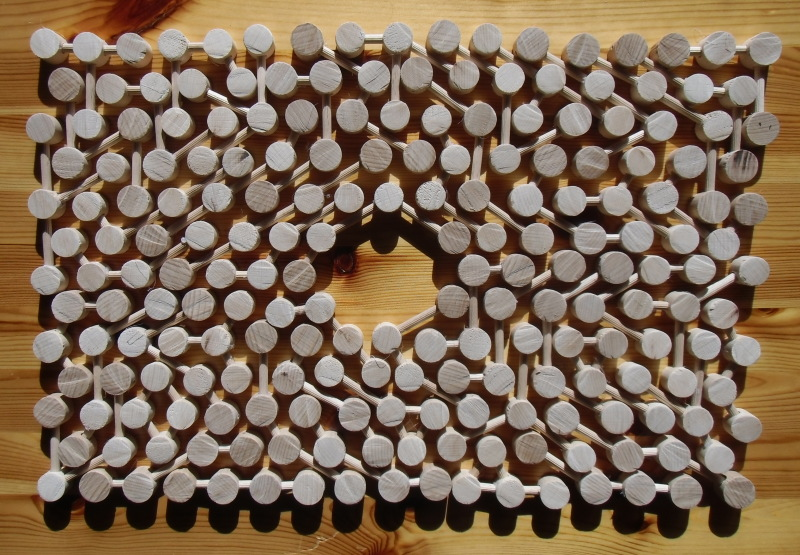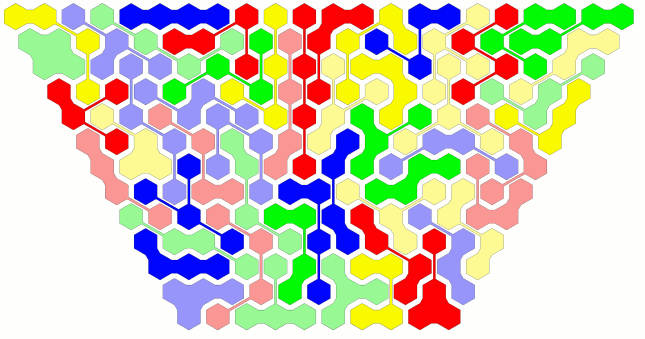Parallelograms are also possible.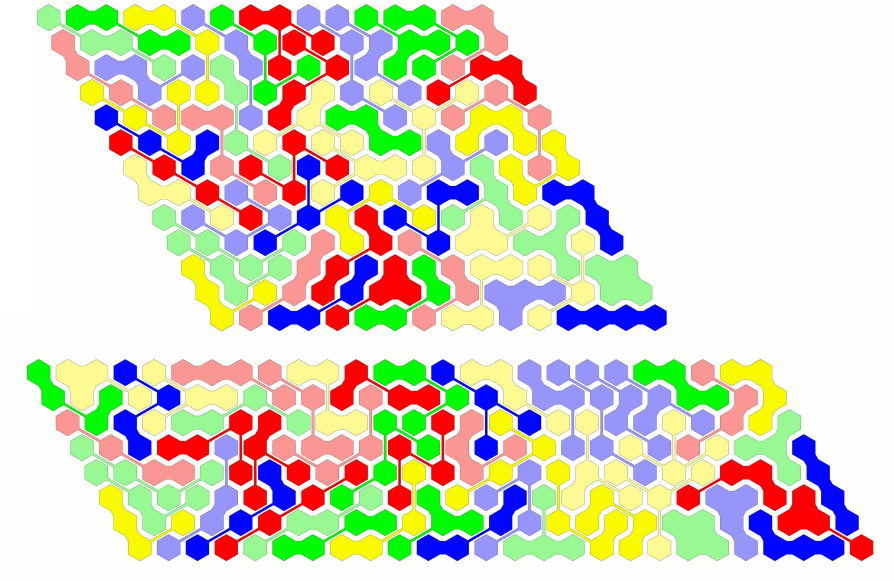For jagged rectangles we need an area of size A=N*L+(N-1)*(L+1) or A=N*L+(N-1)*(L-1). There are some integer solutions for A=208/2=104. Therefore I tried to construct pairs of those rectangles.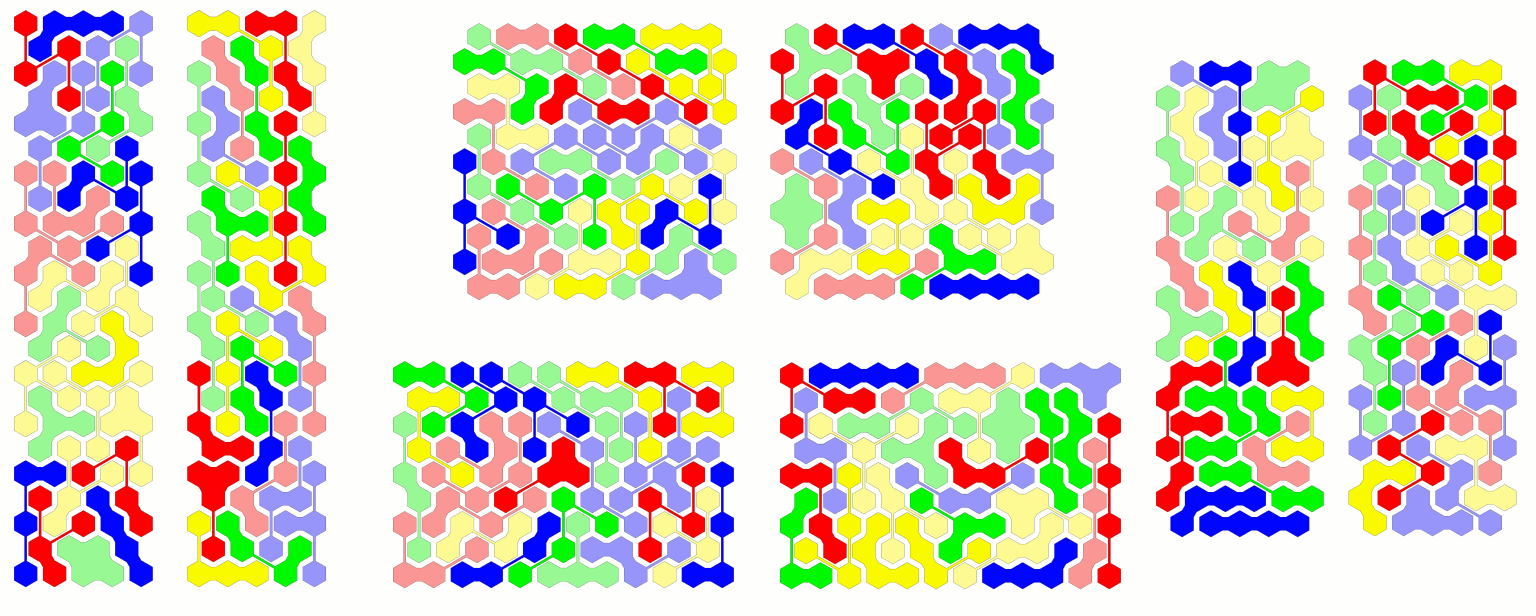At least I wanted to get four congruent symmetric figures. The solution for the berry was found rather fast, because the border is more jagged than that of the rectangle.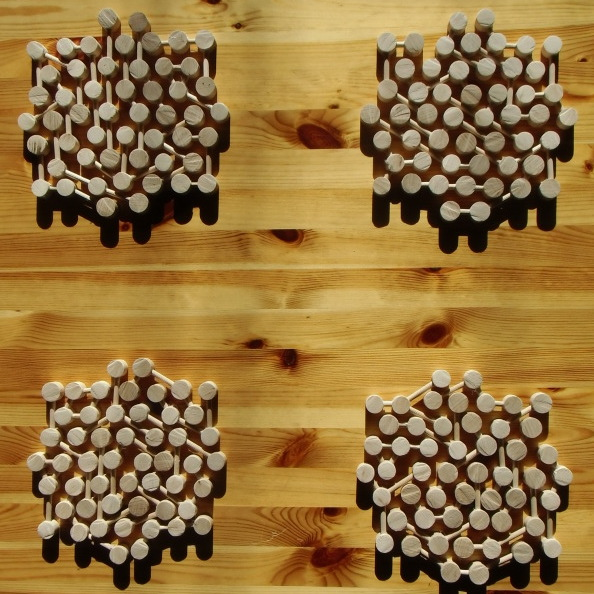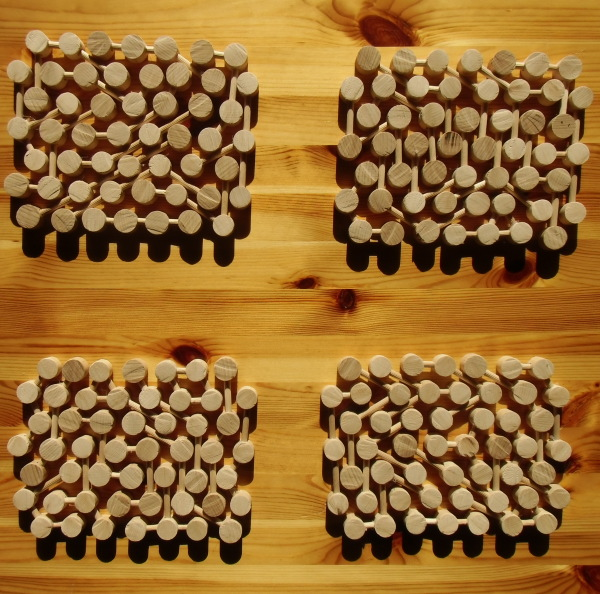## One-sided Rounded Tetrahexes

Here is the set of 89 one-sided rounded tetrahexes with a total area of 356.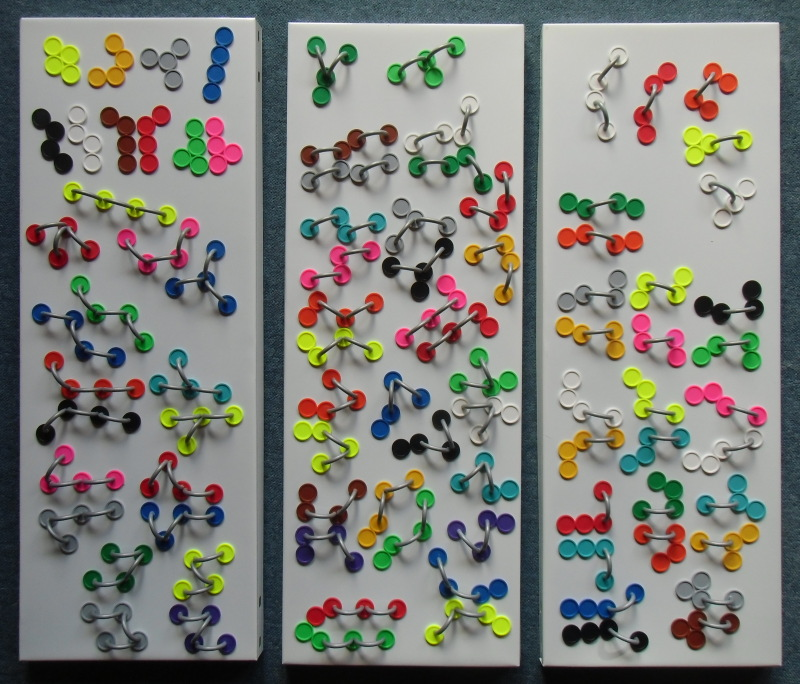Since 356 mod 3 = 2 and 356 mod 6 =2 it's impossible to cover figures with hexagon or triangle symmetry. But we can construct hexagons with 180 degree rotational symmetry.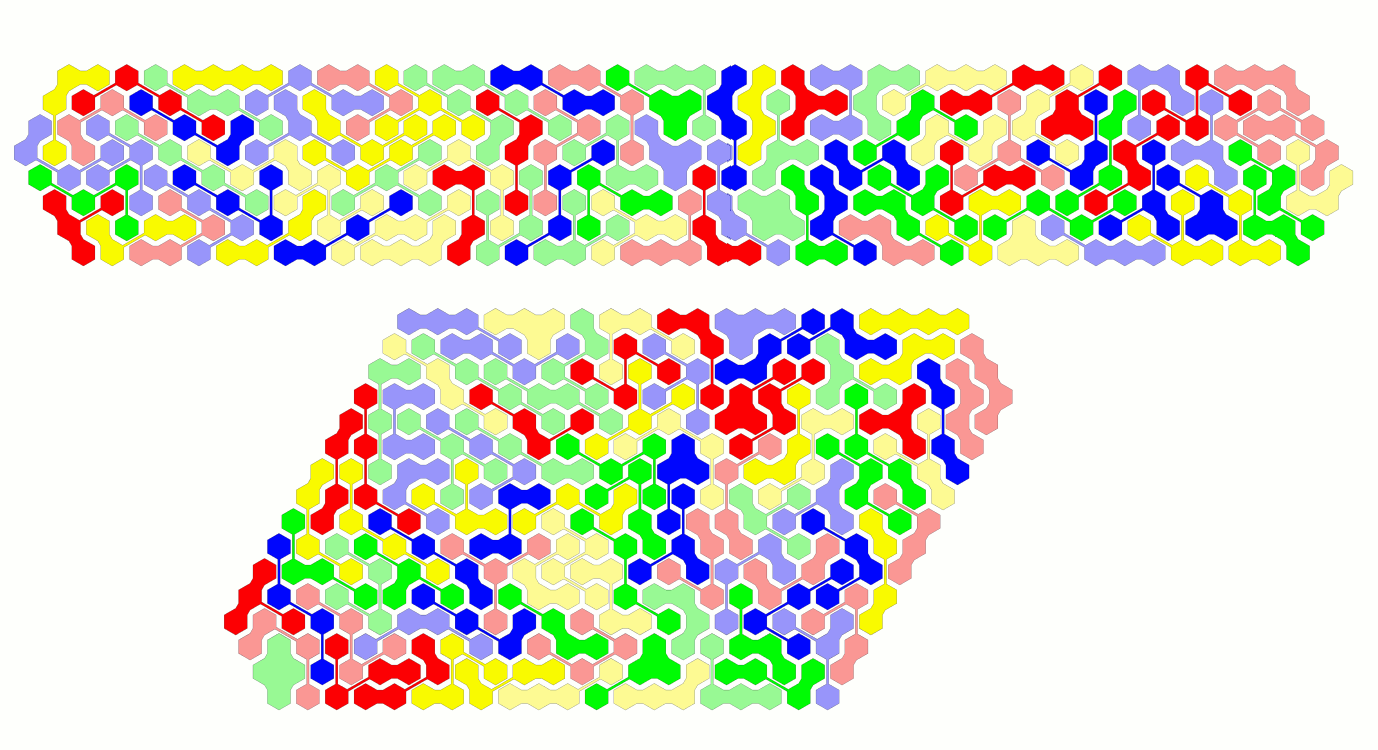A hexagonal ring with two axes of symmetry is also possible.Since 356=256+100 I also looked for two rhombs of size 16 and 10.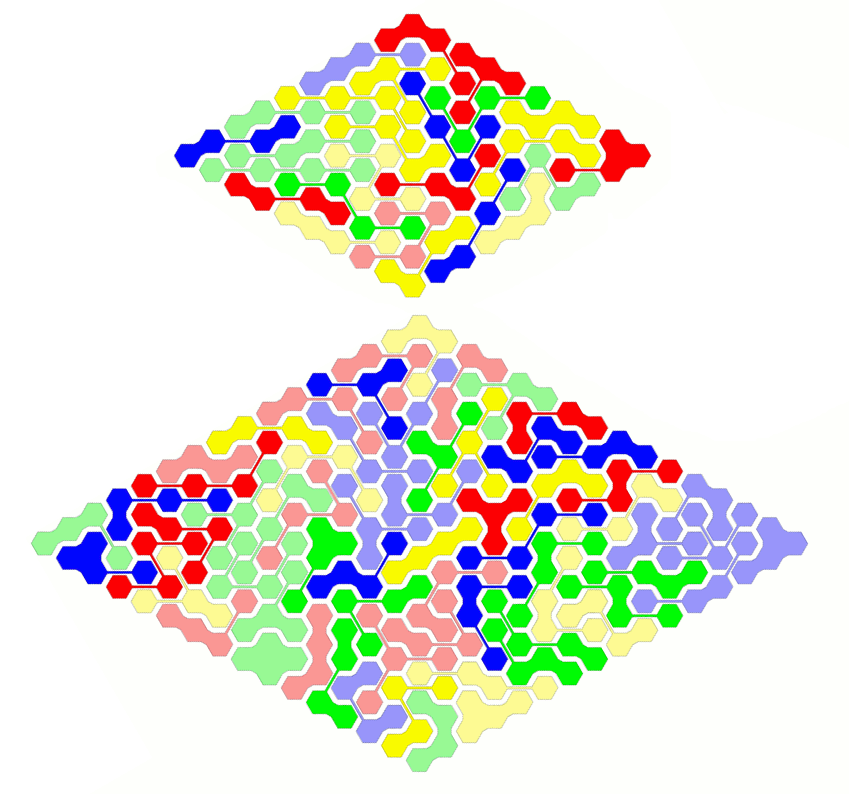I found four different kind of rectangles: 5*40+4*39, 40*5+39*4, 16*11+15*12 and 12*15+11*16.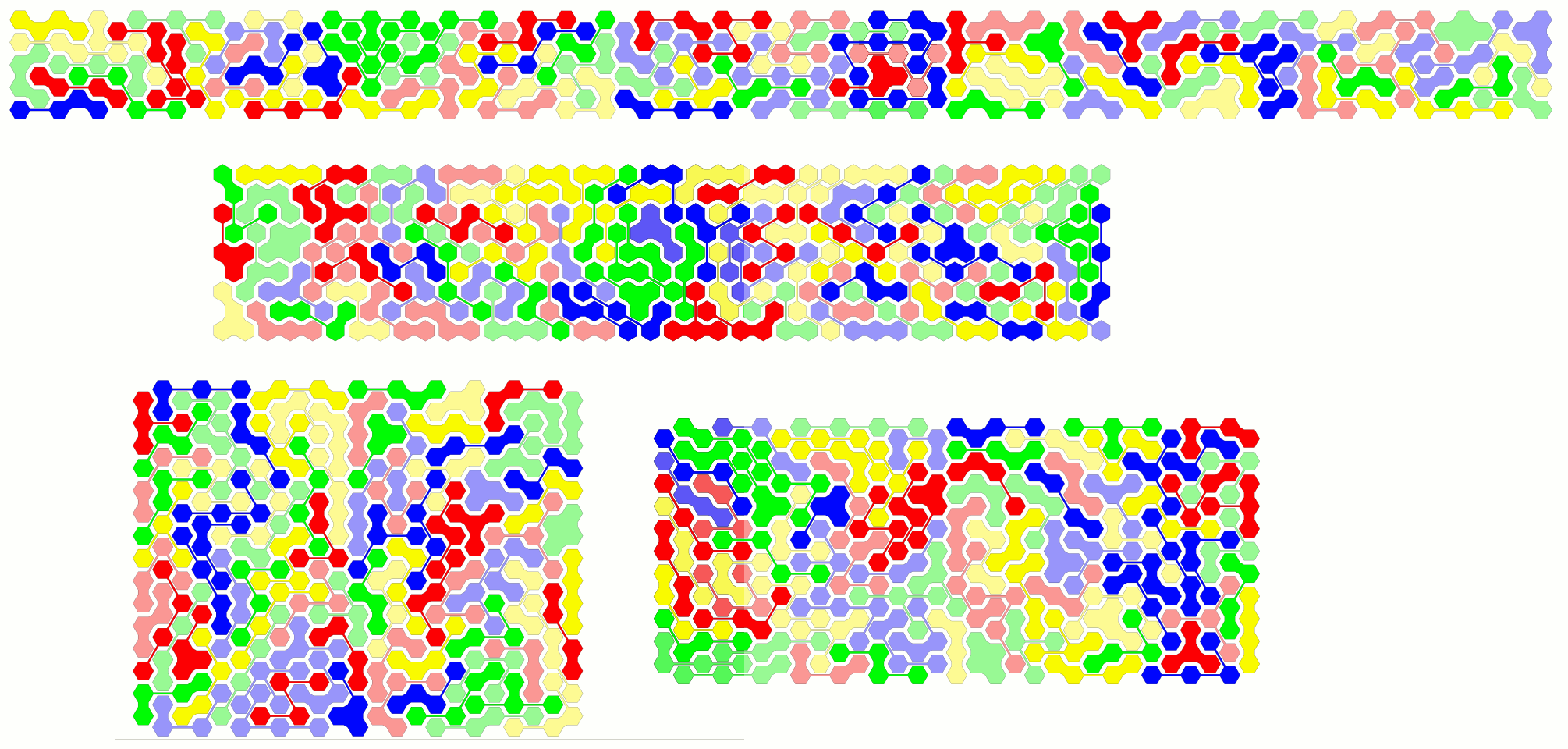A construction with the real pieces confirmed the computer solution.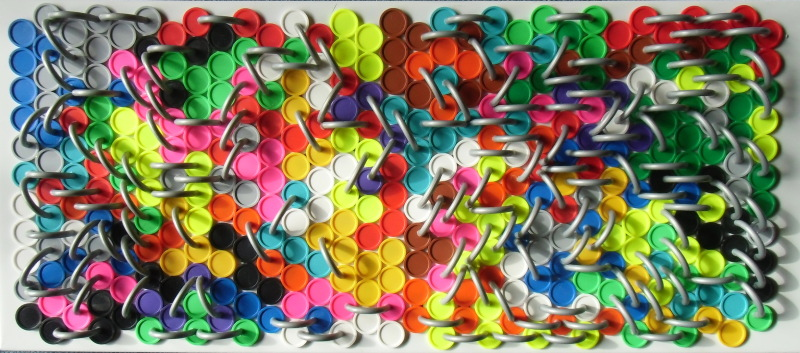Back
Home